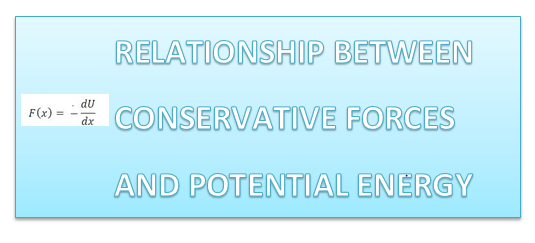# How to find the force if you know the potential energy[latexpage]

Any conservative force acting on an object within a system equals the negative derivative of the potential energy of the system with respect to x.

For one dimensional motion, the force can be found from Potential energy using following formula

$F_{x}=-\frac{\partial U}{\partial x}$

The generalized equation in three dimension is

$F_{x}=-\frac{\partial U}{\partial x}$

$F_{y}=-\frac{\partial U}{\partial y}$

$F_{z}=-\frac{\partial U}{\partial z}$

In vector form

$\boldsymbol{\mathbf{F}}=F_{x}\mathbf{i}+F_{y}\mathbf{j}+F_{z}\mathbf{k}$

Two examples to check on these
1) Spring :
In the case of the deformed spring

$U=\frac{1}{2}Kx^{2}$

Now

$F_{x}=-\frac{\partial U}{\partial x}$

or

$F_{x}=-kx$

Which we already know is the restoring force in Spring mass system

2)  Gravity

$U=mgH$

Now

$F_{x}=-\frac{\partial U}{\partial x}$

or

$F_{x}=-mg$

Which we already know is the gravitational force in gravity

Related articles on energy problems

Equilibrium and Potential energy

How to solve Work And Energy/kinetic energy problems

How to apply law of conservation of energy in mechanics

### Leave a Reply

This site uses Akismet to reduce spam. Learn how your comment data is processed.

Subscribe
Notify of